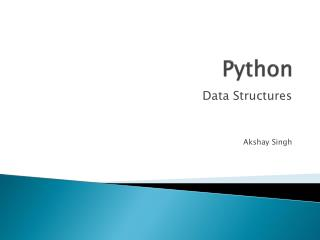DownloadDownload PresentationPython

# Python

Télécharger la présentation## Python

- - - - - - - - - - - - - - - - - - - - - - - - - - - E N D - - - - - - - - - - - - - - - - - - - - - - - - - - -
##### Presentation Transcript

1. Python Data Structures Akshay Singh

2. List • Lists in python can contain any data type • Declaring a list: • a = [‘random’,’variable’, 1, 2]

3. List methods • List.append(x) – adds “x” to the end of the list • List.extend(L) – adds the list “L” to the end of the list • List.insert(I,x) – adds the “I” item to position “x” in the list. • List.remove(x) – removes the first occurrence of “x” from the list.

4. List Methods • List.pop(I) – remove the element at the “I” position and return it. Removes last element if left blank. • List.index(x) – Returns the position of the first occurrence of “x” • List.count(x) – Returns the number of times “x” appears in the list.

5. List Methods • List.sort() – sorts the list. • List.reverse() – reverses the arrangement of elements in the list.

6. Lists as Stacks • Last element in is the first element out. • Use append and pop functions.

7. Lists as Queues • First element in the list is the first the leave. • Use list.append(x) to add to the list and list.popleft() to remove the first element.

8. Functional Programming Tools – filter() • Filter() – returns items from a sequence where the specified function is satisfied. • def f(x): return x % 2 != 0 and x % 3 != 0 • filter(f, range(2, 25)) Output: [5, 7, 11, 13, 17, 19, 23]

9. Functional Programming Tools – map() • Map() – uses the sequence as arguments for the function and returns the results. • def cube(x): return x*x*x • map(cube, range(1, 11)) Output: [1, 8, 27, 64, 125, 216, 343, 512, 729, 1000]

10. Functional Programming Tools – reduce() • Reduce() – Sends the first two elements of the sequence to the function, then the result and the third element and so on. • def add(x,y): return x+y • reduce(add, range(1, 11)) Output: 55

11. List Comprehensions • Easy way to work with lists. • Similar to loops. • vec= [2, 4, 6] • [3*x for x in vec] Output: [6, 12, 18]

12. Function – del() • The del() statement can be used to remove an element from a list. • It does not return a value. • del a : deletes first element in list “a” • del a[2:4] : deletes third and fourth element • del a[:] : deletes all elements in list “a”

13. Tuples • Tuples are a standard sequence data type. • Tuples are a number of values seperated by commas. • Tuples may be nested. • t = 12345, 54321, 'hello!' • u = t, (1, 2, 3, 4, 5) • ((12345, 54321, 'hello!'), (1, 2, 3, 4, 5))

14. Sets • Collection of elements with no duplicates • Can test for membership of elements • basket = [‘a', ‘o', 'a’, ‘p', ‘o', ‘b'] • fruit = set(basket) • fruit • >>>set([‘o', ‘p', ‘a', ‘b']) • 'orange' in fruit • >>>True

15. Dictionaries • Similar to hash tables. • A set of key:value pairs. • tel = {'jack': 4098, 'sape': 4139} • tel.keys() • >>> ['jack‘,’sape’]

16. Looping: iteritems() • Retrieves keys and values from a dictionary. • knights = {'gallahad': 'the pure', 'robin': 'the brave'} • for k, v in knights.iteritems(): • print k, v • >>>gallahadthe pure robin the brave

17. Looping: enumerate() • While looping over a sequence, provides the position of elements. • for i, v in enumerate(['tic', 'tac', 'toe']): • print i, v • >>>0 tic • >>>1 tac • >>>2 toe

18. Looping (contd.) • Zip(list1, list2) : loops 2 or possibly more sequences at the same time. • Reversed(list) : loops over the list in a reverse order. • Sorted(list) : returns the list in a sorted order, however leaves the original list unsorted.

19. Questions?